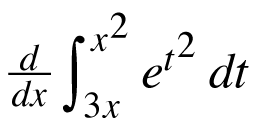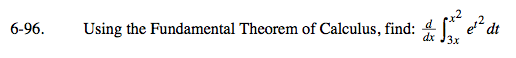### Home > CALC > Chapter 6 > Lesson 6.3.2 > Problem6-96

6-96.

Using the Fundamental Theorem of Calculus, find:. Homework Help ✎This expression is NOT equal to e(x4)e(3x)2.
Explain why not.

If you did not know about the Fundamental Theorem of Calculus,
you would probably evaluate this expression by doing the following steps:
Step 1: Integrate.
Step 2: Differentiate.
As a result of step 1, you would have plugged x2 and 3x into the antiderivative, creating composite functions...
so when you got to step 2, you would have to use the Chain Rule.

2xe(x4) − 3e(3x)2.
Notice that the Chain Rule was applied to each bound.